# The Size and Shape of a Polymer Molecule

Information kindly provided by Gregory Russell, University of Canterbury.

## What are the size and shape of a polymer molecule consisting of N bonds of length b?

The maximum possible mathematical length is that of the fully stretched chain and is Nb, as shown in the figure below. This is called the contour length of the chain.This shape requires that all bond angles are 180°. However, most polymer molecules have backbones consisting of C–C bonds, and so the bond angles are very close to those of a tetrahedron (109.5°). Thus the physically maximum possible length of a polymer chain is that of its so-called zig-zag conformation, shown below:From trigonometry, the length of such a chain is$L_{zig-zag} = Nbsin(\tau/2)$

Using b = 1.54 Å  (for C–C) and  τ= 111.5° (for C–C–C),1 this equation gives Lzig-zag = N x 1.273 Å.

For example, a chain of degree of polymerization DP = 1 000 ⇒ N = 2 000 ⇒ Lzig-zag = 255 nm. This is much larger than polymer chains are measured to be in size. Why? Because rotation of backbone bonds means1 that the above is only one of countless possible
conformations available to a polymer chain. 1 The shape is not exactly tetrahedral because carbon atoms repel each other slightly more than they do hydrogen atoms.

For example, the figure below shows 6 possible conformations for a chain with N = 30:Clearly the zig-zag conformation is statistically unlikely: polymer chains are smaller in size and coiled in shape.

The Random Walk Model
It turns out that a better model for the size and shape of a polymer is that of a random walk. This is a famous problem in mathematics and is  sometimes called the drunken sailor model. It considers that a polymer chain (or drunken sailor) takes steps at random. For example, in one-dimension the movement can be either backwards or forwards at random:

b ← Origin → +b

We want to know RN, the net distance moved – from origin to walk-end – in N steps (see the figure below). Assume that we know RN–1. Then the next (Nth) step must be either forward by b or backward by b, i.e.,$R_N = R_{N-1} + b$ or$R_{N-1} - b$$R_{N}^2 = (R_{N-1}^2 + 2bR_{N-1} + b^2$ or$(R_{N-1})^2 - 2bR_{N-1} + b^2$

Both these values of RN2 are equally likely, because the step may be forwards or backwards with equal probability. Thus the mean squared value of$R_N$ is just the arithmetic mean of the above two possible values of$R_{N}^2$, i.e.,$\langle R_{N}^2 \rangle = \langle R_{N-1}^2 \rangle + b^2$

But$\langle R_{1}^2 \rangle = b^2$ (see picture above), hence$\langle R_{2}^2 \rangle = b^2 + b^2 = 2b^2$, and so on. Thus$\langle R_{N}^2\rangle^0.5 = Nb^2$ and so the root mean squared (rms) end-to-end length of the polymer chain is$\langle R_{N}^2 \rangle ^{0.5} = N^{0.5}b$

This of course is much smaller than the fully extended length, which in one-dimension is Nb (see above). For example, for N = 2 000 the rms length is smaller than the fully extended length by a factor of (2 000)0.5 = 44.7.

The above derivation is for one-dimension only, but of course polymer chains are three-dimensional, as shown below: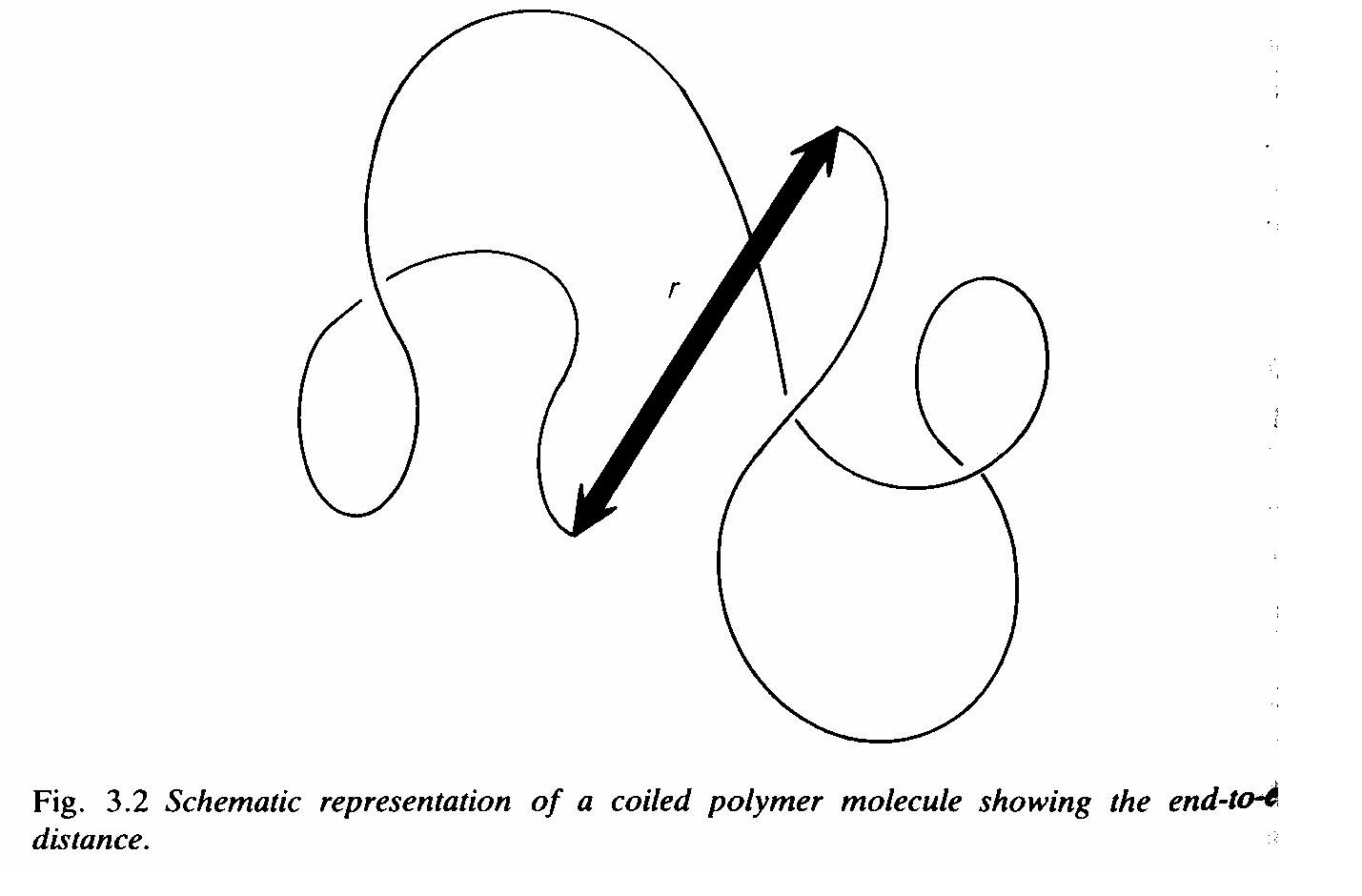To handle the problem in 3 dimensions, we represent the random flight (i.e., polymer chain) as a set of vectors: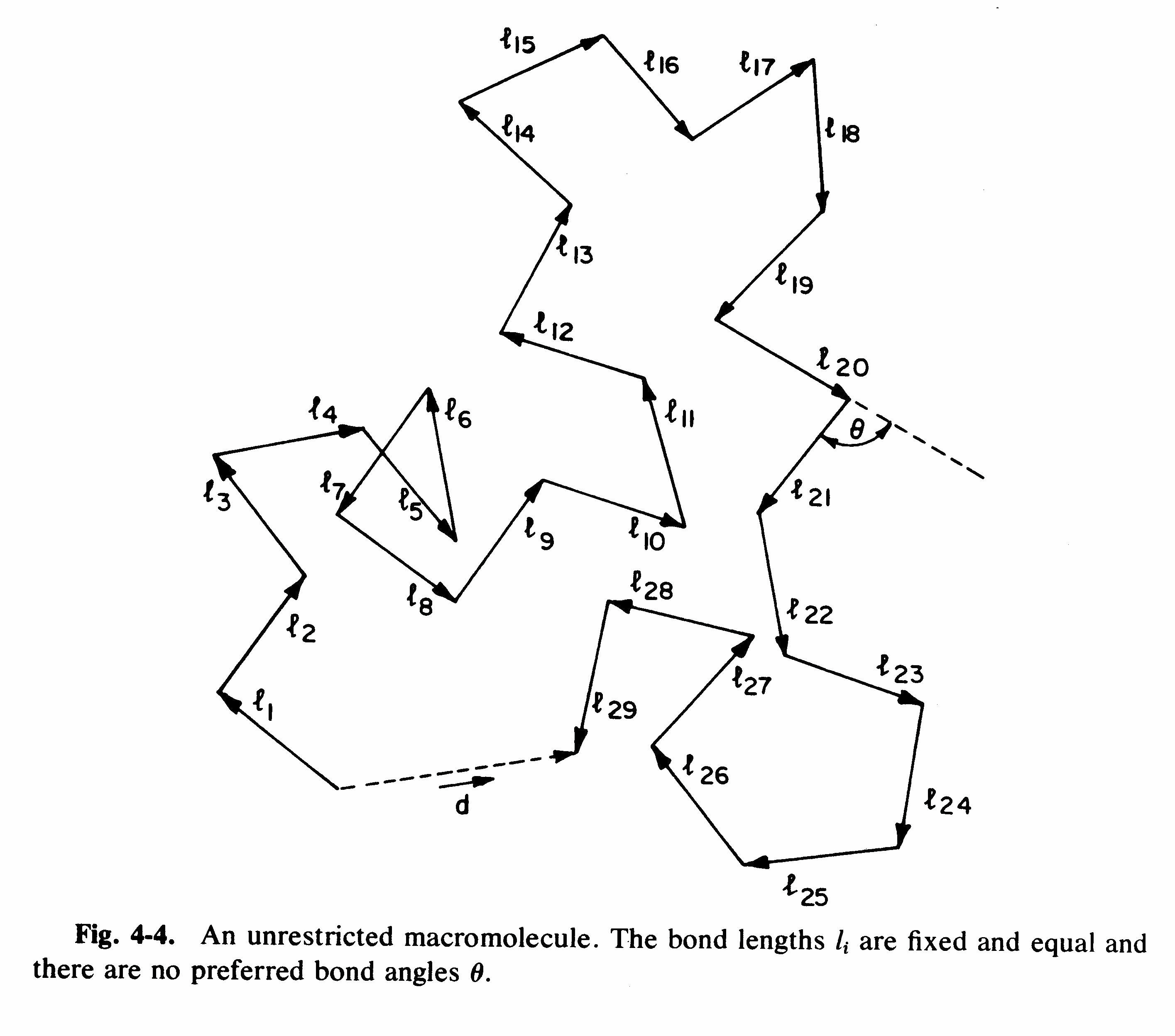Thus the vector R (≡ d in the above diagram) representing the whole chain is:

R = l1 + l2 + … + lN

The squared end-to-end length of the chain is given by the dot product of R with itself, i.e.,$R \cdot R = \left ( \sum_{i=1}^{N}{l_i} \right ) \cdot \left ( \sum_{i=1}^{N}{l_i} \right )$

Now, the dot product of two vectors is given by:$\textbf{a}\cdot\textbf{b} = \textit{ab}cos\theta$

where a is the length of vector a, b the length of b, and θ is the angle between the two vectors (see diagram above). So:$\textbf{l}_i \cdot \textbf{l}_i = b^2$

For different bonds (i.e., i ≠ j), it follows from the definition of a random flight that the value of θ is equally likely to be any value (see figure above).
Averaged over all possible conformations one has (i ≠ j)$\langle \textbf{l}_i \cdot \textbf{l}_j \rangle = \langle l_i l_j cos \theta \rangle = \langle l_i l_j cos \theta \rangle = b^2 \langle cos \theta \rangle = 0$

This is because where all θ are possible and equally likely, the average value of cosθ is zero. Hence$\langle \textbf{R} \cdot \textbf{R} \rangle = \langle R^2 \rangle = Nb^2$

Thus the one-dimensional result holds also in three dimensions.

It is important to remember that$\langle R^2 \rangle ^{0.5} = N^{0.5}b$ is only an average result. At any one time, different polymer chains will have different sizes, and the one polymer chain will change its size over time as its conformation changes.

What is the distribution of sizes?
Consider one end of a polymer chain to be fixed at the origin, as depicted below: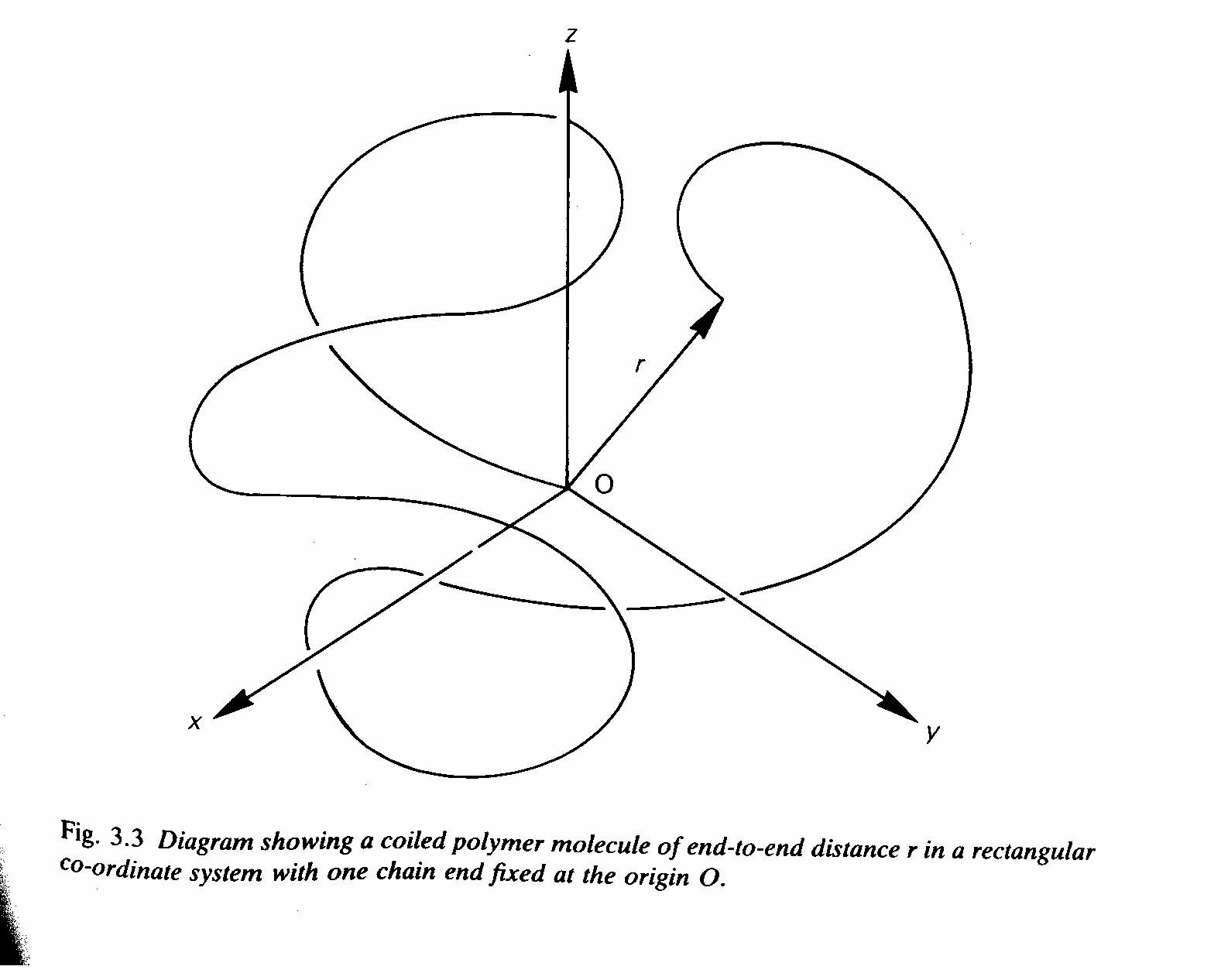It can be shown that:$W(x,y,z) = \left (\beta / \pi^{0.5} \right )^3 \exp(-\beta^2R^2)$, where$\beta = \left [3/(2Nb^2) \right ]^{0.5}$

This gives the probability density W, i.e., the probability of finding the chain end in a small volume dxdydz a distance R from the origin. Shown below is an evaluation of this function for N = 2 000 and b = 0.154 nm.This gives the probability of finding the chain in one small volume element a distance R from the origin. As the value of R increases, the number of such elements a distance R from the origin increases. The probability of finding the chain end at a distance R in any direction from the origin is given by:$W(R) = 4\pi R^2 W(x,y,z)$

This is evaluated below, and one now sees that the maximum probability is some distance from the origin.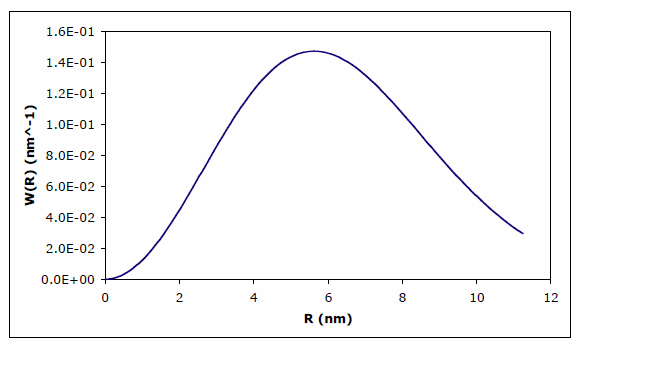The mean squared end-to-end distance is given by:$\langle R^2 \rangle = \frac{{\int_{0}^{\infty}R^2W(R)dR}}{{\int_{0}^{\infty}W(R)dR}}$

Because W(R) is already normalized, one only needs to evaluate the top line of the equation, obtaining$\langle R^2 \rangle = 3/(2 \beta^2) = Nb^2$

This proves that the Gaussian distribution for W correctly gives the random flight result for rms end-to-end distance. However, one notices that W is finite for R > Nb, which of course is physically impossible. This reminds us that these models of polymer chains are not perfect descriptions of reality, even if they are very good ones.
One may similarly evaluate$\langle R \rangle$, the mean value of R. For a Gaussian distribution one obtains$\langle R \rangle = 0.921N^{0.05}b$, a result not as mathematically neat as that for$\langle R^2 \rangle^{0.5}$.

Random Walk Restrictions
Bond Angles
The most obvious way in which polymer chains are not freely jointed is that bond angles are fixed rather than being free. Introducing the restriction of a fixed bond angle τ (see second figure in these notes), it can be shown that:$\langle R^2 \rangle = Nb^2 \frac{1-cos\tau}{1+cos\tau}$

Note that$\langle R^2 \rangle$ ~ N, i.e., the chain can still be represented as a random walk, it’s just that the size of each ‘step’ in the walk is no longer equal to the bond length. Specifically, for the case of C–C backbones, tetrahedral geometry means that$\tau \approx 109.5$°. Since cos(109.5°) = –1/3, one obtains that$\langle R^2 \rangle = 2NB^2$. Qualitatively this is as expected, because τ > 90° must lead to a larger chain compared with random bond angles.

Restricted Bond Rotations
The next way in which polymer chains are not freely jointed is that bond rotation is not completely free. The easiest way to see this is to consider n-butane. This is a specific model for a 3-bond backbone sequence of polyethylene, and it can be considered to be qualitatively representative for any polymer chain:If all 3-bond sequences have the trans conformation above, then the chain will have the zig-zag conformation of the second figure of these notes. But if bond rotation is possible as shown, then a multitude of conformations becomes accessible, and the chain is like a random flight. Thus one sees that bond rotation is the essential ingredient in giving polymer molecules the shape of a random coil.

Clearly bond rotation is not completely free, because steric interactions render the cis conformation above less stable than the trans conformation. In fact the situation is even more complicated than the above 2-state model: there is a bond rotation potential which has minima corresponding to staggered conformations and maxima corresponding to eclipsed conformations, all as illustrated below (here the bond rotation angle is set to φ = 0 for the trans conformation):

To good approximation all 3-bond sequences of a polymer chain will exist as either t, g+ or g–, an effect that clearly acts to extend dimensions (see the first n-butane picture above). In fact taking account of restricted bond rotations in polymers is even more complicated than this. To see this,
consider the possible conformations of n-pentane:As shown, the g+g– and gg+ conformations bring the methyl end groups into close proximity, and thus make these conformations of higher energy (lower probability) than would be deduced on the basis of the 3-bond potential alone. These are termed interdependent steric restrictions, and clearly they act to extend chain dimensions still further. Of course matters are more complicated still when the polymer chain has sidegroups (as is usual), e.g. phenyl groups on every second carbon in the case of polystyrene. Such side groups obviously tend to further hinder bond rotations and introduce additional interdependent steric interactions involving the side groups.

It is possible to take all the above effects into account theoretically. Because the results are very complicated in the usual case of sidegroups being involved, it is commonly just written that:$\langle R^2 \rangle = Nb^2 \frac{1-cos\tau}{1+cos\tau}\sigma^2$

The new parameter σ is termed the steric parameter. Thus the random-flight nature is retained, but restricted bond rotations considerably expand a polymer chain: typically σ is 1.6–2.3. For tetrahedral backbones (see above), one has$\langle R^2 \rangle^{0.5} = N^{0.5}b2^{0.5}\sigma$

Thus rms end-to-end distances of real polymer chains are typically greater than those of so-called freely-jointed chains by factors of 2–3$(\approx 2^{0.5}\sigma)$.

Excluded Volume
Up until now we have been considering only short-range interactions. The random-flight model assumes there are no long-range interactions: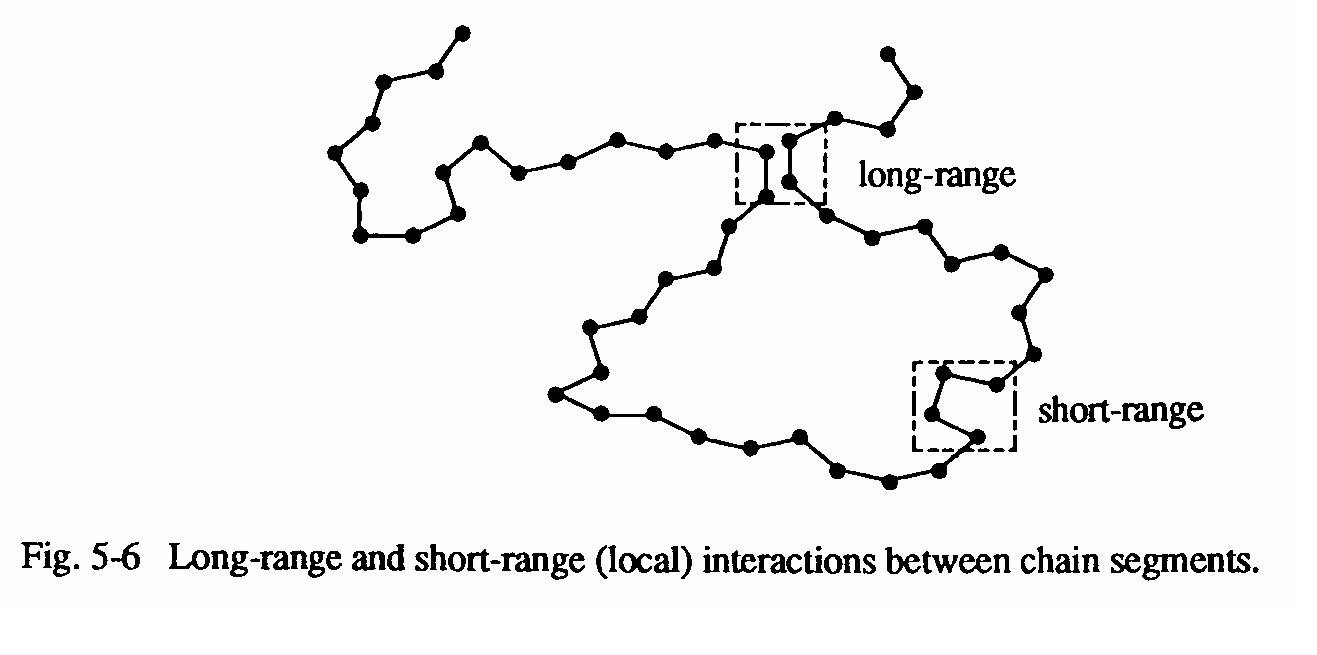The most obvious long-range interaction is that a chain must avoid itself: two chain segments cannot be in the same place at the same time. This is termed excluded volume: rather than a polymer chain being a random walk it is a self avoiding walk (SAW).
Quite obviously this must act to expand chain dimensions, but accounting for it precisely is a tremendously complicated exercise. It is possible to show that:$\left (\langle R^2 \rangle_{SAW} \right )^{0.5} \sim N^{3/(d+2)}$
where d is the dimensionality of the space.

Thus for a 1-dimensional walk$(d=1)$,$\left (\langle R^2 \rangle_{SAW} \right )^{0.5} \sim N$. This is illustrated in the figure below: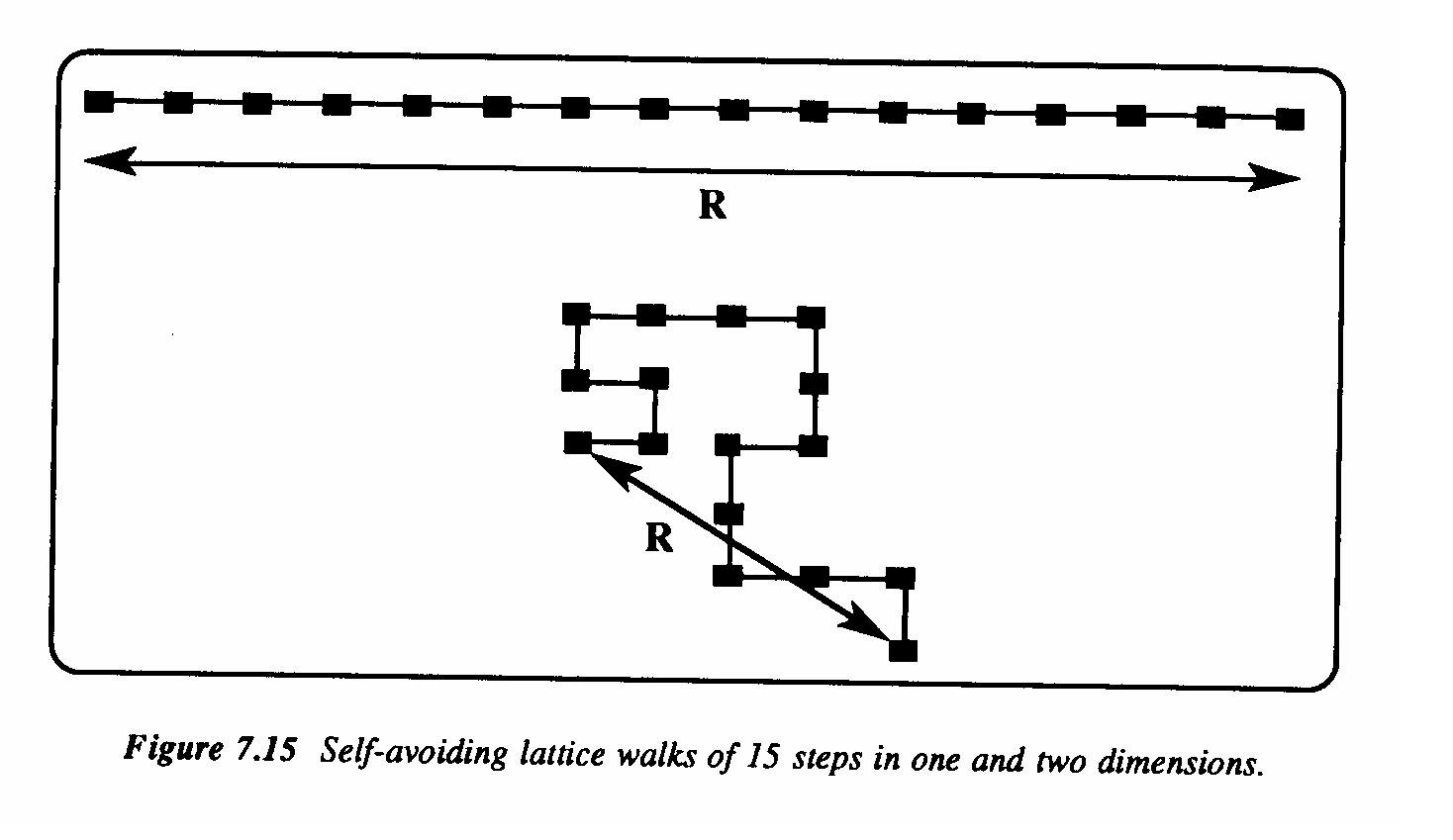For 3-dimensional SAWs, corresponding to real polymer chains, one has that$\left (\langle R^2 \rangle_{SAW} \right )^{0.5} \sim N^{0.6}$ (cf.$\langle R^2 \rangle_{0.5} \sim N^{0.5}$ where crossability is allowed). So self-avoidance does indeed expand end-to-end distance.

Solvent Effects
No consideration has yet been paid to the environment of a polymer chain, which has implicitly been assumed to be a vacuum. In reality a polymer chain will be surrounded by solvent. If segment-solvent interactions are more favourable than solvent-solvent and segment-segment interactions, then each polymer segment will prefer to be surrounded by solvent molecules rather than be near other polymer segments. The solvent is said to be a good solvent for the polymer, and clearly this situation must result in expansion of the polymer chain (e.g. consider the 2-dimensional SAW shown above).

Conversely, for a poor solvent the polymer chains contract in order to reduce the number of segment-solvent contacts. These effects are extremely complicated to treat mathematically, but there is a simple end result:$\langle R^2 \rangle_{0.5} \sim N_{\alpha}$

where α ≈ 0.5–0.6, depending on solvent quality: α ≈ 0.5 for a poor solvent, α ≈ 0.6 for a good solvent. Note that this includes also the effect of excluded volume.

Because it already acts to reduce segment-segment contacts, there is little further expansion where solvent-segment interactions are enthalpically favourable.

Summary
What are the size and shape of a polymer molecule? This is arguably the most interesting, challenging and beautiful question that has been answered about polymers:
1. The shape is that of a random coil.
2. The size (rms end-to-end distance) is given by$\langle R^2 \rangle_{0.5} \sim N_{\alpha}$, where α ≈ 0.5–0.6.
Here is a picture of what it all looks like: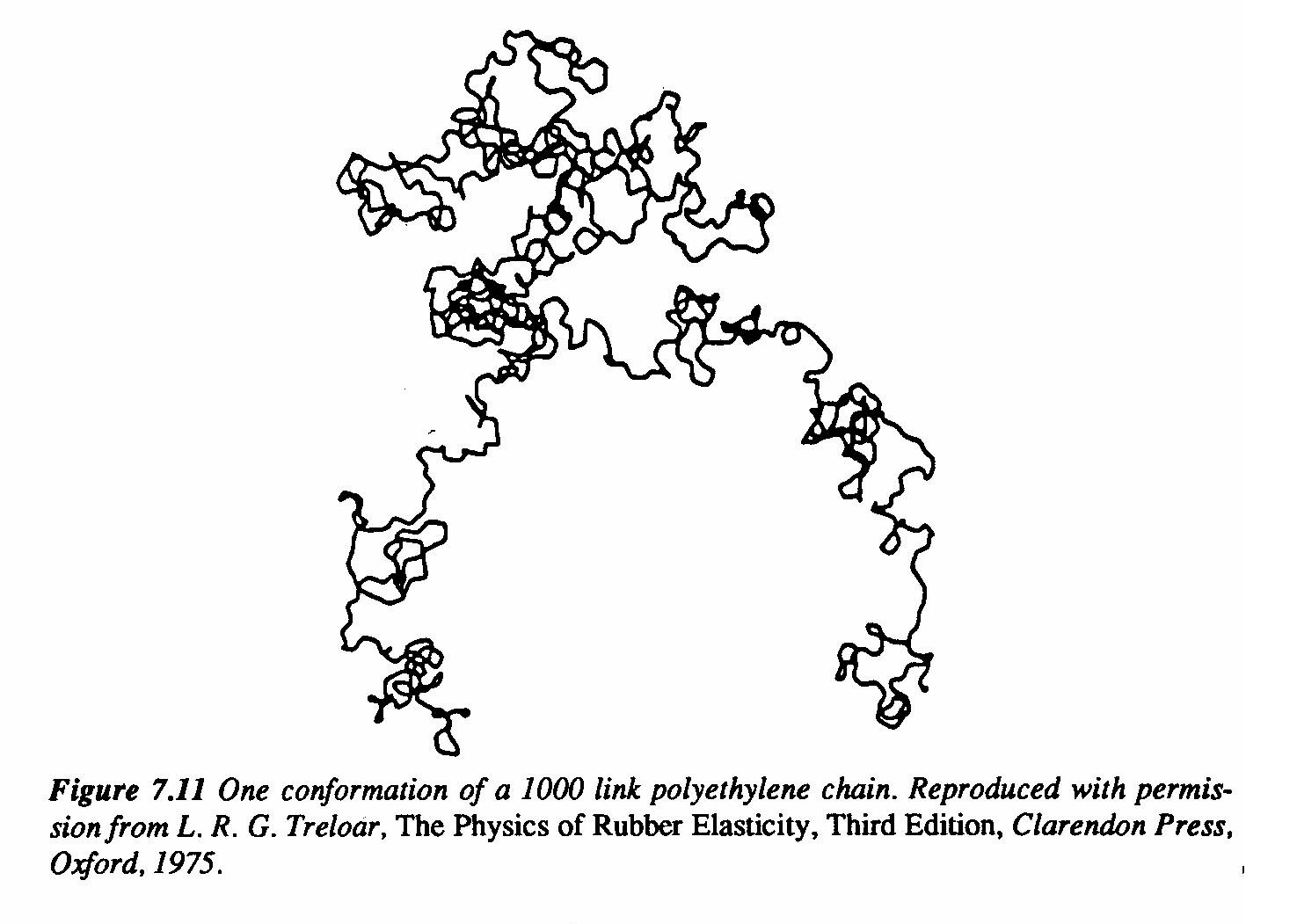There are experimental means for determining polymer shape and measuring polymer size. These verify all the results above.